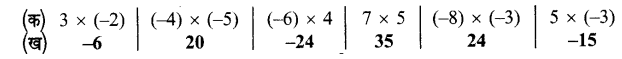• +91 9971497814
• info@interviewmaterial.com

# Chapter 3- पूर्णांक Ex-3.4 Interview Questions Answers

### Related Subjects

Question 1 :
निम्नांकित के मान ज्ञात कीजिए (ज्ञात करके)-
(क) 8 x (4) x (-5)
(ख) (-10) x (-10) x (-10)
(ग) (-2) x 35 x (-5)
(घ) (-8) x (+57) x 0
(ङ) (-1) x (-2) x (-3) x (4)
(च) (4) x (8) x (-12) x (-5)

(क) 8 x (4) x (-5) = 160
(ख) (-10) x (-10) x (-10) = -1000
(ग) (-2) x 35 x (-5) = 350
(घ) (-8) x (+57) x 0 = 0
(ङ) (-1) x (-2) x (-3) x (4) = 24
(च) (4) x (8) x (-12) x (-5) = 1920

Question 2 :
निम्नांकित के मान ज्ञात कीजिए –
(क) (-7) x (0) x (-57) x (-57)
(ख) 1456 x 625 – 456 x 625
(ग) (-187) x (-54) + (-187) x (46)
(घ) 18764 x 99 – (-18764)
(ङ) 15341 x (-2) + (-15341) x 98
(च) (-8) x {10 – 5 – 43 + 98}

(क) (-7) x (0) x (-57) x (-57)

(ख) 1456 x 625 – 456 x 625 = 0
= 625 (1456 – 456)
= 625 x 1000
= 625000

(ग) (-187) x (-54) + (-187) x (-46)
= -187[-54 + (46)]
= -187 x (-100)
= 18700

(घ) 18764 x 99 – (-18764)
= 18764 [99 – (-1)]
= 18764 x 100
= 1876400

(ङ) 15341 x (-2) + (-15341) x 98
= 15341 [-2 + (-98)]
= 15341 x (-100)
= -1534100

(च) (-8) x {10-5-43 + 98}
= (8) x 60 = 480

Question 3 : पंक्ति ‘क’ में दो पूर्णांकों का गुणनफल पंक्ति ‘ख’ में दिये गये हैं। इन्हें मिलान कर लिखिए। (सही मिलान करके)-Question 4 :
उस पूर्णांक को ज्ञात कीजिए जिसका -1 से गुणनफल है-
(क) -37
(ख) 49
(ग) 0

(क) -37 ÷ (-1) = 37
(ख) 49 ÷ (-1) = -49
(ग) 0 ÷ (-1) = 0

Question 5 :
उत्तर के चार विकल्पों में से सही उत्तर बताइए-
(क) (3 + 2) x 7 का मान है।
(i) 3 + 2 x 7
(ii) 3 x 7 + 2
(iii) 3 x 7 + 2 x 7
(iv) 3 x 7 – 2 x 7

(ख) (3 – 2) x 6 का मान हैः
(i) 3 x 6 – 2 x 6
(ii) 3 x 6 – 2
(iii) 3 x 6 + 2 x 6
(iv) 3 x 2 x 6

(ग) (-8) x (-5) का मान है।
(i) -40
(ii) 40
(iii) 13
(iv) उपर्युक्त में कोई नहीं।

(घ) तीन ऋणात्मक पूर्णांकों के गुणनफल में एक धनात्मक पूर्णांक से गुणा करने पर गुणनफल का चिहुन है-
(i) धनात्मक
(ii) न तो धनात्मक और न ऋणात्मक
(iii) ऋणात्मक
(iv) उपर्युक्त में से कोई नहीं।

(क) (iii) 3 x 7 + 2 x 7
(ख) (i) 3 x 6 – 2 x 6
(ग) (ii) 40
(घ) (iii) ऋणात्मक

Question 6 :
निम्नलिखित में सत्य या असत्य बताइए (सत्य-असत्य छाँटकर)-
(i) 5 ऋणात्मक पूर्णाकों का सतत गुणनफल धनात्मक होता है।
(ii) दो ऋणात्मक पूर्णांकों का गुणनफल धनात्मक होता है।
(iii) दो पूर्णांकों में यदि केवल एक ऋणात्मक है, तो उनको गुणनफल ऋणात्मक होता है।
(iv) -17 का विपरीत +17 है।
(v) किसी पूर्णांक का विपरीत ज्ञात करने के लिए उसमें शून्य से गुणा करते हैं।
(vi) 1 से किसी पूर्णाक में गुणा करने पर गुणनफल वही पूर्णांक होता है।

(i) 5 ऋणात्मक पूर्णाकों का सतत गुणनफल धनात्मक होता है। (असत्य)
(ii) दो ऋणात्मक पूर्णांकों का गुणनफल धनात्मक होता है। (सत्य)
(iii) दो पूर्णांकों में यदि केवल एक ऋणात्मक है, तो उनको गुणनफल ऋणात्मक होता है। (सत्य)
(iv) -17 का विपरीत +17 है। (सत्य)
(v) किसी पूर्णांक का विपरीत ज्ञात करने के लिए उसमें शून्य से गुणा करते हैं। (असत्य)
(vi) 1 से किसी पूर्णाक में गुणा करने पर गुणनफल वही पूर्णांक होता है। (सत्य)

Todays Deals### Chapter 3- पूर्णांक Ex-3.4 Contributorskrishan

Name:
Email:

# Latest News# 9000 interview questions in different categories